# C语言_干翻操作符_含义+结合性+优先级+例子分析（上）

hackernew

0收藏

1，（ ）操作符

eg: test()操作数是函数名test。
test(x,y)操作数是test ,x,y（函数名+内部的传参数）

eg: sizeof()的括号可以省略，说明sizeof不是函数，是一种操作符。

（强制转化类型）

``````#include <stdio.h>
int main()
{
int a =(int)3.14 ;
printf("a=%d ",a);
return 0;
}
``````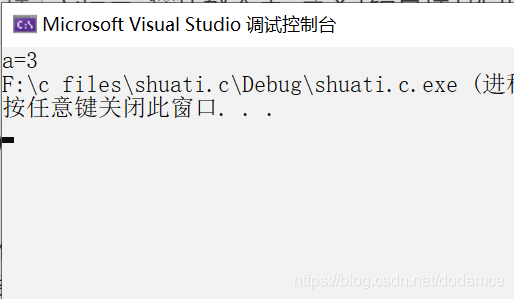2，[ ]操作符

eg:
arr 操作数为arr和4。

3，->操作符

eg：

``````#include<stdio.h>
struct student
{
//结构体的成员
char name;
int age;
char sex;
};
int main()
{
//结构体访问操作符是建立在结构体上的
struct student s = { "小红",18,"woman" };//初始化结构体
struct student* ps = &s;
/*printf("%s",(*ps).name);*/
printf("%s",ps->name);
return 0;
}

``````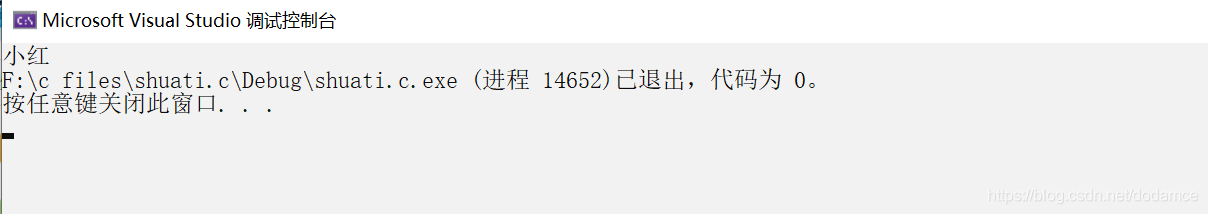4， . 操作符

eg:

``````#include<stdio.h>
struct student
{
//结构体的成员
char name;
int age;
char sex;
};
int main()
{
//结构体访问操作符是建立在结构体上的
struct student s = { "小红",18,"woman" };//初始化结构体
printf("%s",s.name);
return 0;
}

``````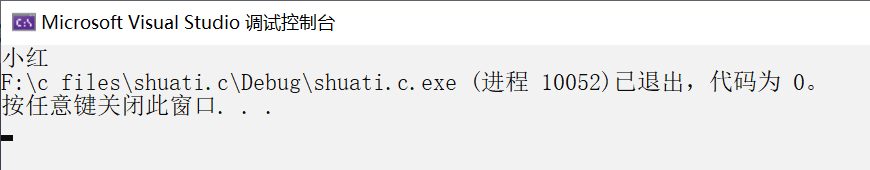``````#include<stdio.h>
struct student
{
//结构体的成员
char name;
int age;
char sex;
};
int main()
{
//结构体访问操作符是建立在结构体上的
struct student s = { "小红",18,"woman" };//初始化结构体
struct student* ps = &s;
printf("%s",(*ps).name);
return 0;
}
``````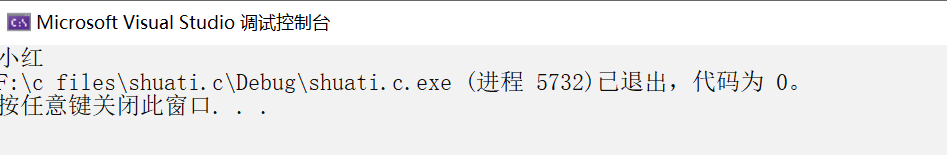1，！操作符

``````#include<stdio.h>
int main()
{
int a = 0;
int b = 6;
printf("a=%d b=%d",!a,!b);
return 0;
}
``````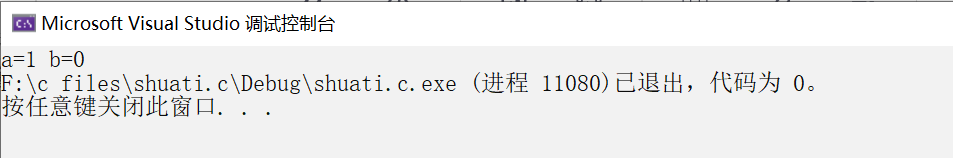``````#include <stdio.h>

int main()
{
int x = 0;
while (scanf("%d", &x) != EOF)
{
if (x >= 140)
printf("Genius\n");
else
printf("Not Genius\n");
}
return 0;
}

``````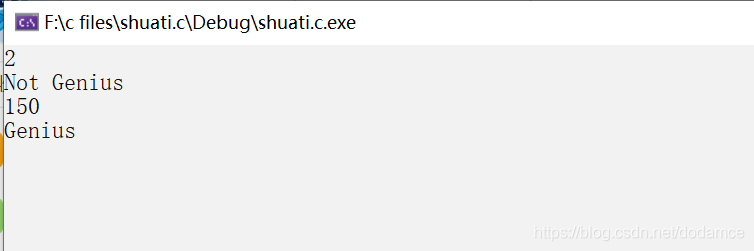(文件读取到最后返回一个EOF,EOF本质是-1为真，！EOF为0，程序就会停下来来实现多组输入)

``````#include <stdio.h>
int main()
{
int input = 0;
if (!input)//如果input为假执行
{
//....
}
if (input)//如果input为真执行
{
//....
}
return 0;
}
``````

2, ~操作符

（按二进制位取反）

-----------------------------------------------------------------

eg（都是32位）

10000000000000000000000000000001表示-1

00000000000000000000000000000001表示1

eg:
1 1 1 1 1…1 1(32位)（符号位1说明是负数）补码

1 1 1 1 1…1 0(32位)（补码-1到反码）

1 0 0 0 0…0 1(32位)（符号位不变其余位按位取反到原码）

-----------------------------------------------------------------

-1取反为32个0是0

0在计算机会判断为假

``````int main()
{
int x = 0;
while (~scanf("%d", &x) )
{
if (x >= 140)
printf("Genius\n");
else
printf("Not Genius\n");
}
return 0;
}
``````

3，++操作符

``````#include <stdio.h>
int main()
{
int a = 10;
int b = ++a;
printf("a=%d b=%d",a,b);
return 0;
}
``````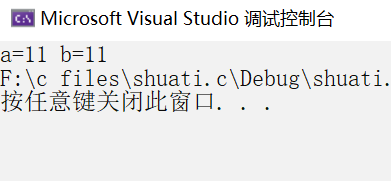``````#include <stdio.h>
int main()
{
int a = 10;
printf("a=%d ",++a);
return 0;
}``````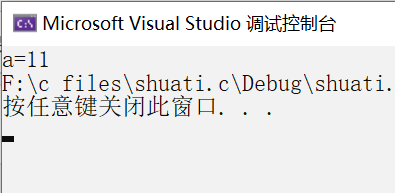``````#include <stdio.h>
int main()
{
int a = 10;
printf("a=%d ",++a);
return 0;
}
````````````#include <stdio.h>
int main()
{
int a = 10;
int b = a++;
printf("a=%d b=%d",a,b);
return 0;
}
``````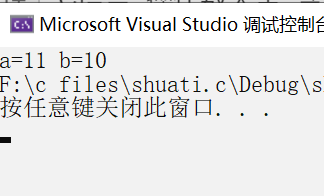``````#include <stdio.h>
int main()
{
int a = 10;
printf("a=%d ",a++);
return 0;
}
``````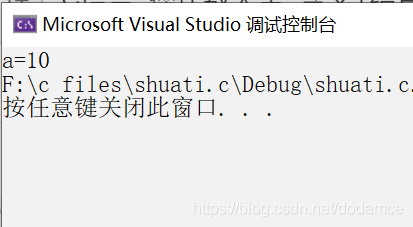4，- -操作符

``````#include <stdio.h>
int main()
{
int a = 10;
int b = --a;
printf("a=%d b=%d",a,b);
return 0;
}
``````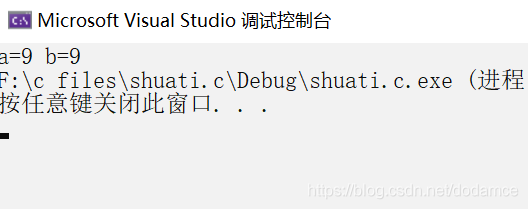``````#include <stdio.h>
int main()
{
int a = 10;

printf("a=%d ",--a);
return 0;
}
``````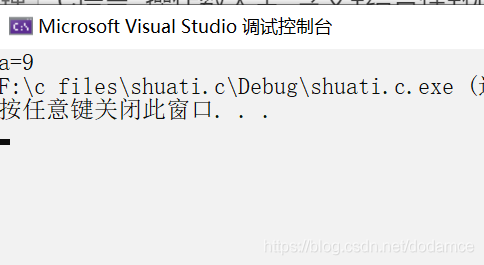``````#include <stdio.h>
int main()
{
int a = 10;
int b = a--;
printf("a=%d b=%d",a,b);
return 0;
}
``````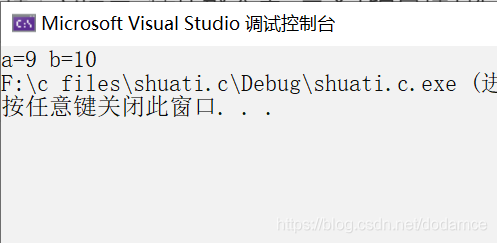``````#include <stdio.h>
int main()
{
int a = 10;
printf("a=%d ",a--);
return 0;
}
``````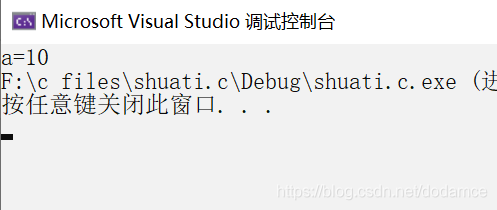5，-符号运算符

（注意负号运算符和减法运算符不同，负号运算符是单目运算符，减法运算符是双目运算符，系统会自动识别）

``````#include <stdio.h>
int main()
{
int a = 10;
a = -a;//负号运算符
a = a - 10;//减法运算符
return 0;
}
``````

6，* （指针）操作符

（具体指针性质在之后提到）

``````#include <stdio.h>
int main()
{
int a = 5;
int* pr = &a;//存放地址
printf("%d",*pr);//找到指针变量所指对象
return 0;
}
``````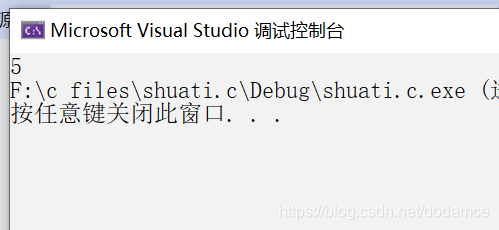7，&（取地址）操作符

8，sizeof 操作符

sizeof可以计算变量大小，也可以计算变量类型大小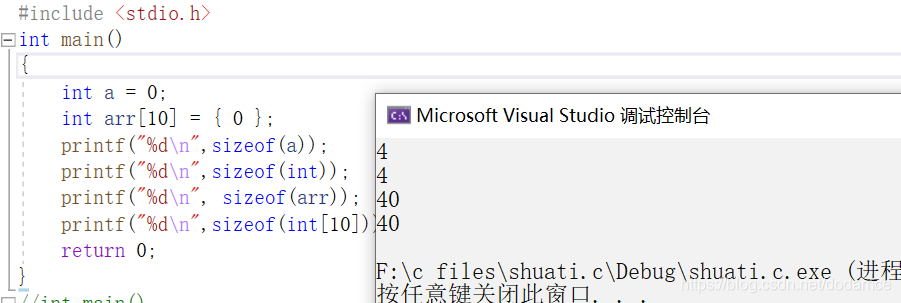（注意int是数组的类型）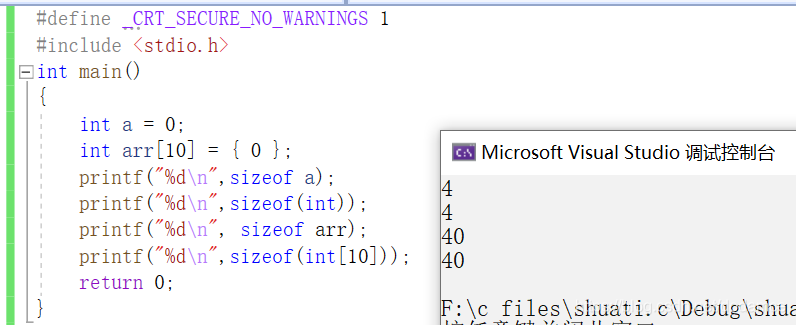sizeof与strlen的区别

sizeof是操作符求大小，strlen是求字符串长度函数其必须有（）函数调用操作符

sizeof会计算\0的大小，而strlen不会计算\0的长度（\0）是字符串结束的标志。

sizeof括号内的表达式是不会执行计算的

eg:

``````#include <stdio.h>
int main()
{
int s = 20;
int a = 10;
printf("%d\n",sizeof(s=a+5));
printf("%d",s);
return 0;
}
``````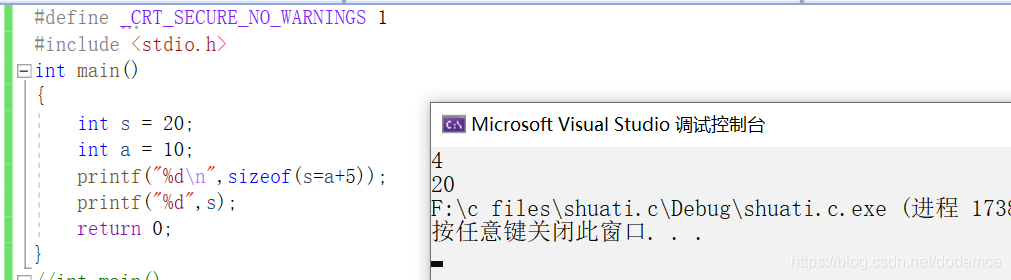（注意这个例子中决定sizeof值的是s的类型不是表达式中a的类型）

1.*（乘法），/，%操作符

*（乘法）操作符 运算不在赘述；

/（除法）操作符
（注意要想通过除法得到一个浮点型，除数与被除数至少有一个要写出小数）

``````#include <stdio.h>
int main()
{
float i = 3 / 2.0;
printf("%f",i);
return 0;
}
``````

%（求余操作符）
（注意%不能有小数）帖子
视频
声望
粉丝
社区精华内容